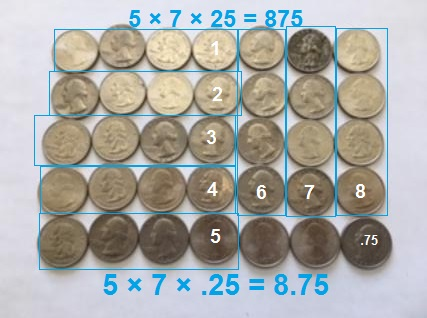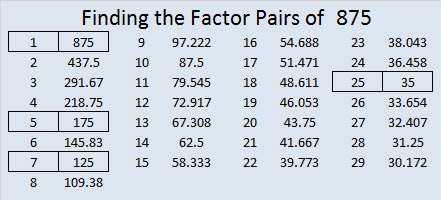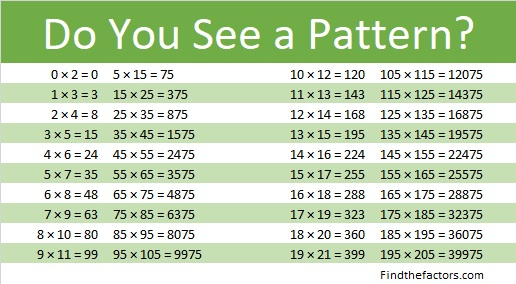# 875 Multiplication and Division Facts

This simple illustration of \$8.75 can be used to show a variety of multiplication facts. We can easily see that this much money can be divided by 5, 7, 25, and even 125.Likewise, if we know that the amount of money is \$8.75, we can write that as 8¾ and do some other easy division problems if we divide the money into groups:

• 8¾ ÷ 1¼ = 7 because 1¼ is 5 quarters.
• 8¾ ÷ 1¾ = 5 because 1¾ is 7 quarters.

Now, it might not be obvious that there really is \$8.75 in money unless we divide the money into groups of four quarters.Since \$8.75 is the same as 8¾, we can try this division problem, too:

How much is 8¾ ÷ 1½?What other fractional division problems can you do with these 35 quarters?

Here’s a little more about the number 875:

875 is the hypotenuse of three Pythagorean triples:

• 525-700-875 which is (3-4-5) times 175.
• 245-840-875 which is (7-24-25) times 35.
• 308-819-875 which is 7 times (44-117-125)

Factors of 875 were shown in red in those triples. Here are all of 875’s factors:

• 875 is a composite number.
• Prime factorization: 875 = 5 × 5 × 5 × 7, which can be written 875 = 5³ × 7
• The exponents in the prime factorization are 3 and 1. Adding one to each and multiplying we get (3 + 1)(1 + 1) = 4 × 2 = 8. Therefore 875 has exactly 8 factors.
• Factors of 875: 1, 5, 7, 25, 35, 125, 175, 875
• Factor pairs: 875 = 1 × 875, 5 × 175, 7 × 125, or 25 × 35
• Taking the factor pair with the largest square number factor, we get √875 = (√25)(√35) = 5√35 ≈ 29.5803989875 is in this cool pattern:This site uses Akismet to reduce spam. Learn how your comment data is processed.